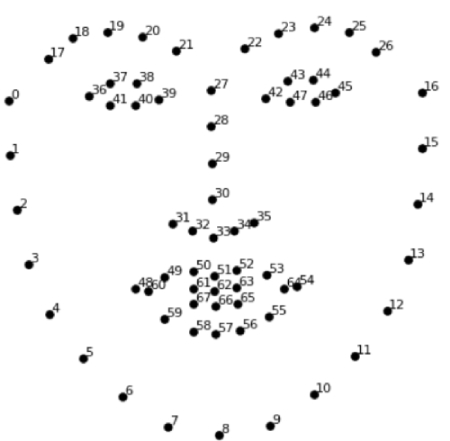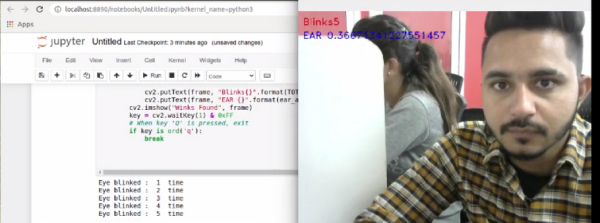• +91-9872993883
• +91-8283824812
• info@ris-ai.com

# Eye Blink Detection with OpenCV, Python and Dlib¶

Here we are going to detect eye blink of a person and keep a count of it.For this we will be learning a library called Dlib which basically has a wide range of machine learning algorithms and tools to create complex softwares but also has amazing features for computer vision and image processing.We will make use of these powerful features to study face patterns and use it to track eye blinks.

The basic idea that Dlib uses is that every face has the same features ie it will have two eyes, one nose, a lip, a jaw line and two eyebrows.So it doesn't really matter what is the origin of a person, these properties are going to be same for every face.Based on this idea, it has localized the regions of a face and put numbers to represent those regions.Below is a diagram that better explains the idea.

Today, we are going to build upon this knowledge and develop a computer vision application that is capable of detecting and counting blinks in video streams using facial landmarks and OpenCV.

## Real-Time Eye Blink Detection Using Facial Landmarks

In :
import numpy as np
import cv2
import dlib
from scipy.spatial import distance as dist

In :
JAWLINE_POINTS = list(range(0, 17))
RIGHT_EYEBROW_POINTS = list(range(17, 22))
LEFT_EYEBROW_POINTS = list(range(22, 27))
NOSE_POINTS = list(range(27, 36))
RIGHT_EYE_POINTS = list(range(36, 42))
LEFT_EYE_POINTS = list(range(42, 48))
MOUTH_OUTLINE_POINTS = list(range(48, 61))
MOUTH_INNER_POINTS = list(range(61, 68))
EYE_AR_THRESH = 0.22
EYE_AR_CONSEC_FRAMES = 3
EAR_AVG = 0
COUNTER = 0
TOTAL = 0


### Understanding the “eye aspect ratio” (EAR)

The eye of a person is given six points which maps the entire eye.These six points are used to calculate EAR which gives a value that estimates whether a person's eye is closed or open.If a person's eye is closed, the value will be low and if it is open, the value will be high.Below is an illustration of the idea.

Once EAR is calculated, we can use this a thershold value and make a condition that any value less that this means the eyes are closed and greater than this means the eyes are open.Now a blink means that an eye is closed for some moment before it opens again.So we can put a further condition that if EAR is below the threshold for three consecutive frames and then the value spikes, it means a blink has taken place.Then we can keep of count of the number of blinks.

At first let is look at how Dlib identifies and assigns the numbers when a face is provided to it.Below is my original photo which I will pass to the Dlib library to identify the facial landmarks.In :
def eye_aspect_ratio(eye):
# compute the euclidean distance between the vertical eye landmarks
A = dist.euclidean(eye, eye)
B = dist.euclidean(eye, eye)
# compute the euclidean distance between the horizontal eye landmarks
C = dist.euclidean(eye, eye)
# compute the EAR
ear = (A + B) / (2 * C)
return ear


We can see it successfully identifies the facial landmarks and highlights them.Now we shall try to detect and count eye blinks of a person in realtime.Below is a gif that implements that feature.

In [ ]:
# to detect the facial region
detector = dlib.get_frontal_face_detector()
predictor = dlib.shape_predictor('shape_predictor_68_face_landmarks.dat')
# capture video from live video stream
cap = cv2.VideoCapture(0)
while True:
# get the frame
#frame = cv2.resize(frame, (0, 0), fx=0.5, fy=0.5)
if ret:
# convert the frame to grayscale
gray = cv2.cvtColor(frame, cv2.COLOR_BGR2GRAY)
rects = detector(gray, 0)
for rect in rects:
x = rect.left()
y = rect.top()
x1 = rect.right()
y1 = rect.bottom()
# get the facial landmarks
landmarks = np.matrix([[p.x, p.y] for p in predictor(frame, rect).parts()])
# get the left eye landmarks
left_eye = landmarks[LEFT_EYE_POINTS]
# get the right eye landmarks
right_eye = landmarks[RIGHT_EYE_POINTS]
# draw contours on the eyes
left_eye_hull = cv2.convexHull(left_eye)
right_eye_hull = cv2.convexHull(right_eye)
# cv2.drawContours(frame, [left_eye_hull], -1, (0, 255, 0), 1) # (image, [contour], all_contours, color, thickness)
# cv2.drawContours(frame, [right_eye_hull], -1, (0, 255, 0), 1)
# compute the EAR for the left eye
ear_left = eye_aspect_ratio(left_eye)
# compute the EAR for the right eye
ear_right = eye_aspect_ratio(right_eye)
# compute the average EAR
ear_avg = (ear_left + ear_right) / 2.0
if ear_avg < EYE_AR_THRESH:
COUNTER += 1
else:
if COUNTER >= EYE_AR_CONSEC_FRAMES:
TOTAL += 1
cv2.drawContours(frame, [left_eye_hull], -1, (0, 255, 0),1)  # (image, [contour], all_contours, color, thickness)
cv2.drawContours(frame, [right_eye_hull], -1, (0, 255, 0), 1)
COUNTER = 0

cv2.putText(frame, "Blinks{}".format(TOTAL), (10, 30), cv2.FONT_HERSHEY_DUPLEX, 0.7, (0, 0, 255), 1)
cv2.putText(frame, "EAR {}".format(ear_avg), (10, 60), cv2.FONT_HERSHEY_DUPLEX, 0.7, (255,0,0), 1)
cv2.imshow("Winks Found", frame)
key = cv2.waitKey(1) & 0xFF
# When key 'Q' is pressed, exit
if key is ord('q'):
breakIn [ ]:
# release all resources
cap.release()
# destroy all windows
cv2.destroyAllWindows()

In [ ]:


In [ ]: